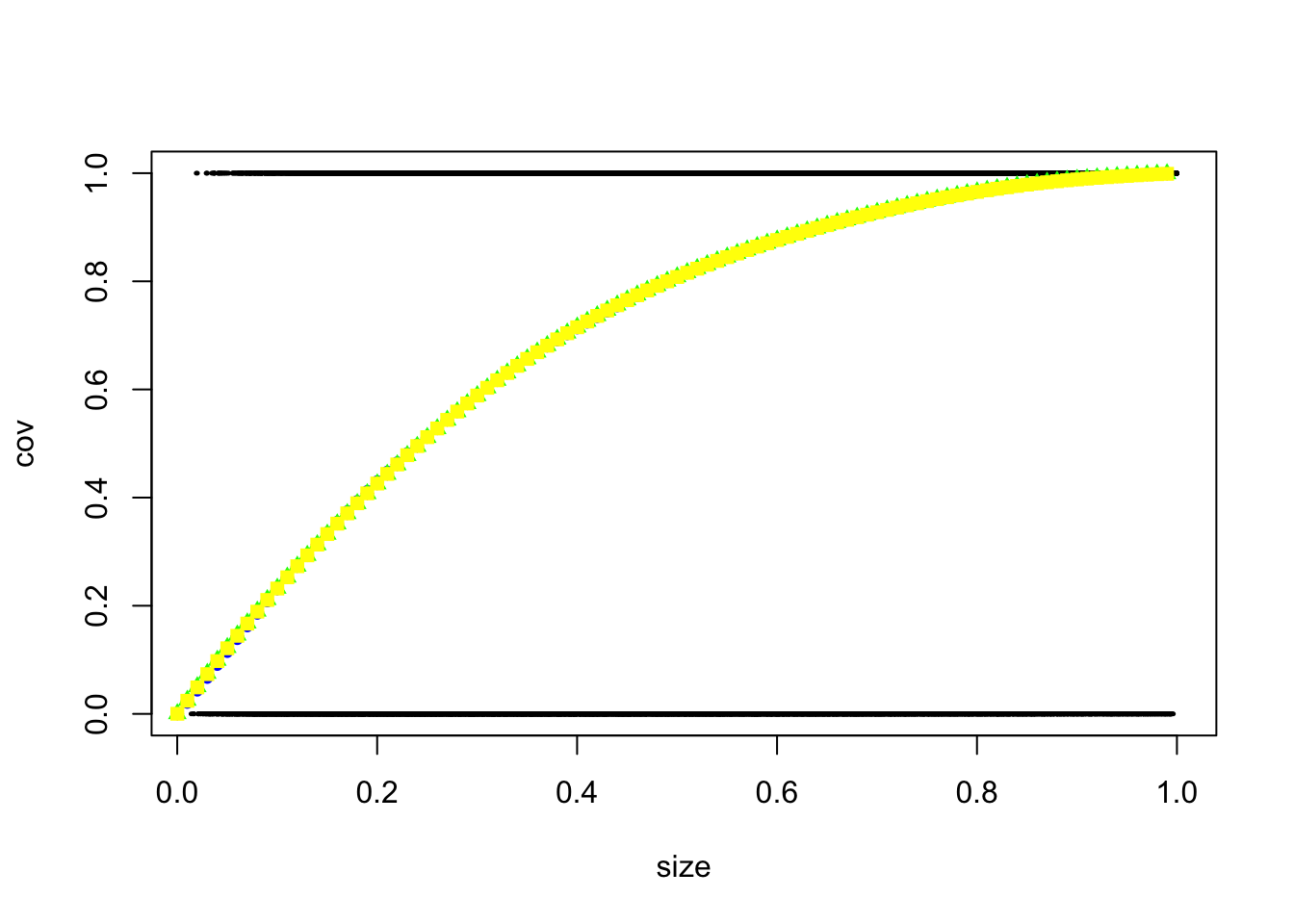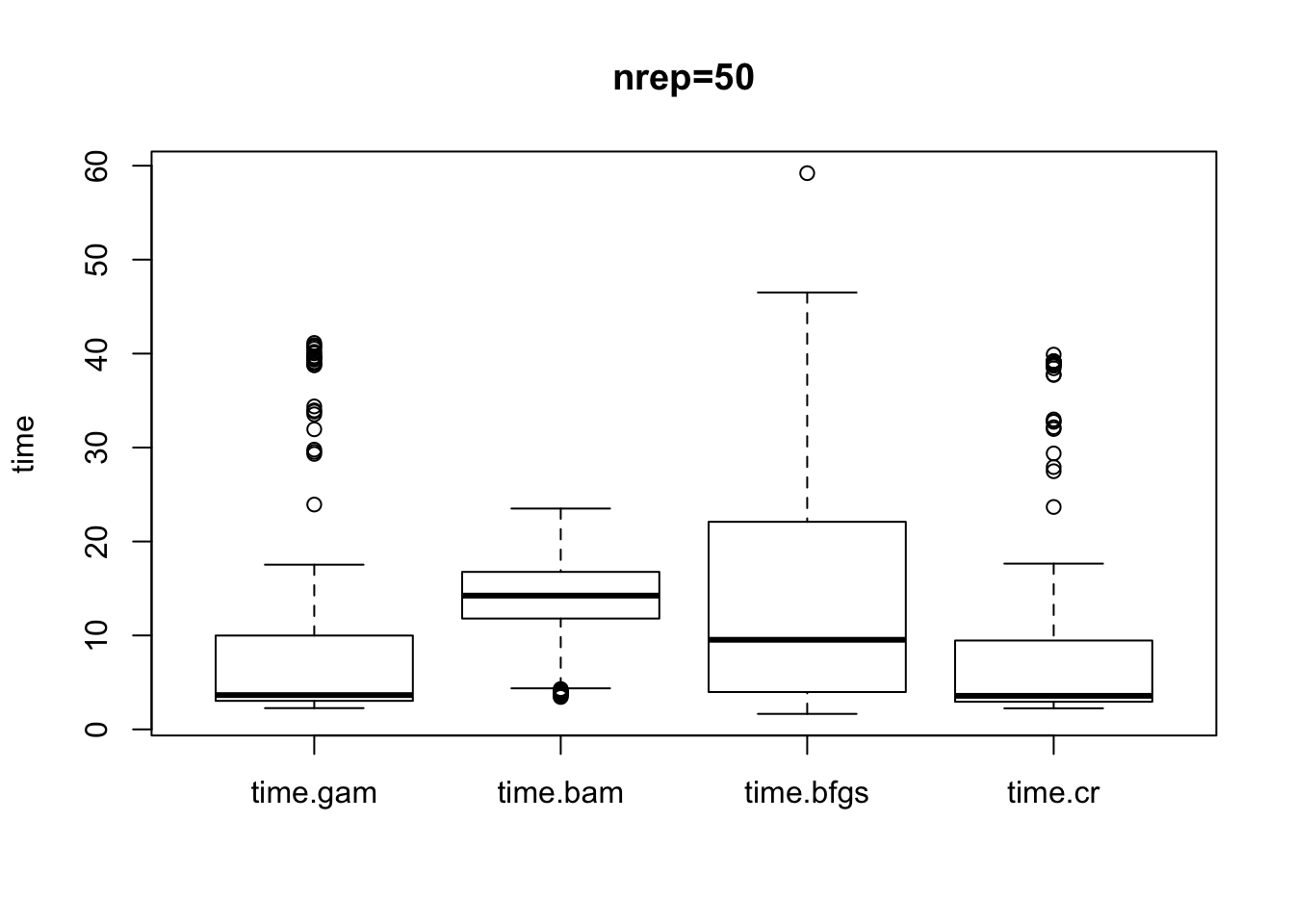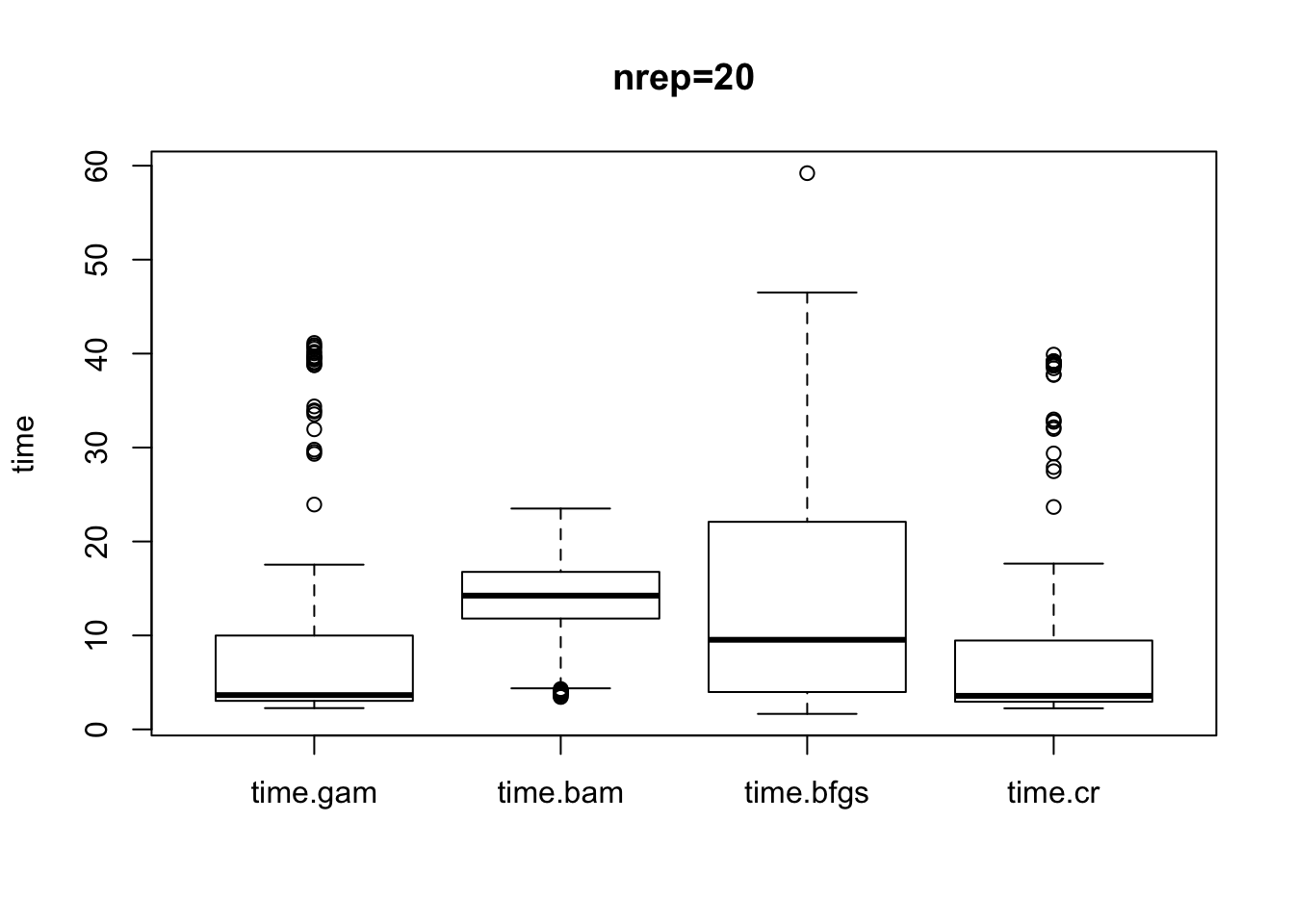## Further Development of Corrected Coverage Method

• If all the user wants to do is to estimate the corrected coverage, then we can just simulate and average the binary covered column of these simulated credible sets.

• If we can fit a model, then we choose a GAM. We tried logistic regression but it wasn’t bendy enough and we don’t have enough data to fit a random forest (these aren’t smooth and need lots of data at specific points on the x axis to calculate the average and to fit a line through - only accurate estimates at these dense x regions).

• So far, our package provides users with an accurate coverage probability. Would be good if we could extend the package such that the user is provided with a new ‘required threshold’ value that they should use in the credset function to obtain a credible set with the required true coverage.

### How often is a GAM actually being fitted?

### corr.maxz0 method
colMeans(res1)
##      good   warning     error
##   0.00000   4.10198 195.84208
### corr.muhat.ave method
colMeans(res2)
##       good    warning      error
##   0.000000   3.264356 196.679703
• I tried to fit a GAM for the simulated credible sets for each SNP considered causal (5000 data points for each GAM). If the GAM could be fit with no problem, then my code returns ‘good’, similarly for ‘warning’/‘error’. The figures above are the colMeans for the simulations. On average 195/200 GAMs fitted (each SNP causal), an error is returned.

• I told my original simulations to just average the covered column is ANY out of the 200 GAMs (each SNP considered causal) returned an error (as these returned NaNs in the final vector and I couldn’t average).

• This means that a GAM wasn’t actually being fit at all! I was just averaging the covered column.

• We didn’t identify this earlier as we were working on lower power scenarios.

Try fitting a GAM to the cumulative pps (rather than the size of the cred set). I.e. get 200 data points for everyone 1 in original method.

BUT for just 100 simulations for each snp causal, that is $$100\times 200\times 200=4e+06$$ data points to fit a gam to which mgcv::gam struggles with. I later explore variations for quicker GAM fitting.

### Fitting GAM to Whole Data

Try fitting a GAM to the cumulative pps (rather than the size of the cred set). I.e. get 200 data points for everyone 1 in original method.

• For each simulated posterior probability system for each variant considered causal a GAM is fitted for covered vrs cpp (cumulative probabilities) where the variants have been sorted into descending order. This GAM is then used to predict the coverage probability at the claimed coverage.

• We could also consider a backwards approach, whereby the corrected target (claimed coverage on x axis) is read off for some target coverage value. For example, if a user wanted a credible set with 90% corrected coverage, then we could tell them that they need to use a ‘required’ threshold value of X. They can then use this value as the threshold parameter in the credsets function to obtain their new credible set with the desired corrected coverage.

• A problem with this is that the data from the fitted GAM often looks like this,

test <- readRDS("/Users/anna/Google Drive/PhD/feb/credsets/check_errors/test.RDS")
test
##      cpp             y
## 1   0.00  0.000000e+00
## 2   0.01  0.000000e+00
## 3   0.02  0.000000e+00
## 4   0.03  0.000000e+00
## 5   0.04  0.000000e+00
## 6   0.05  0.000000e+00
## 7   0.06  0.000000e+00
## 8   0.07  0.000000e+00
## 9   0.08  0.000000e+00
## 10  0.09  0.000000e+00
## 11  0.10  0.000000e+00
## 12  0.11  0.000000e+00
## 13  0.12  0.000000e+00
## 14  0.13  0.000000e+00
## 15  0.14  0.000000e+00
## 16  0.15  0.000000e+00
## 17  0.16  0.000000e+00
## 18  0.17  0.000000e+00
## 19  0.18  0.000000e+00
## 20  0.19  0.000000e+00
## 21  0.20  0.000000e+00
## 22  0.21  0.000000e+00
## 23  0.22  0.000000e+00
## 24  0.23  0.000000e+00
## 25  0.24  0.000000e+00
## 26  0.25  0.000000e+00
## 27  0.26  0.000000e+00
## 28  0.27  0.000000e+00
## 29  0.28  0.000000e+00
## 30  0.29  0.000000e+00
## 31  0.30  0.000000e+00
## 32  0.31  0.000000e+00
## 33  0.32  0.000000e+00
## 34  0.33  0.000000e+00
## 35  0.34  0.000000e+00
## 36  0.35  0.000000e+00
## 37  0.36  0.000000e+00
## 38  0.37  0.000000e+00
## 39  0.38  0.000000e+00
## 40  0.39  0.000000e+00
## 41  0.40  0.000000e+00
## 42  0.41  0.000000e+00
## 43  0.42  0.000000e+00
## 44  0.43  0.000000e+00
## 45  0.44  0.000000e+00
## 46  0.45  0.000000e+00
## 47  0.46  0.000000e+00
## 48  0.47  0.000000e+00
## 49  0.48  0.000000e+00
## 50  0.49  0.000000e+00
## 51  0.50  0.000000e+00
## 52  0.51  0.000000e+00
## 53  0.52  0.000000e+00
## 54  0.53  0.000000e+00
## 55  0.54  0.000000e+00
## 56  0.55  0.000000e+00
## 57  0.56  0.000000e+00
## 58  0.57  0.000000e+00
## 59  0.58  0.000000e+00
## 60  0.59  0.000000e+00
## 61  0.60  0.000000e+00
## 62  0.61  0.000000e+00
## 63  0.62  0.000000e+00
## 64  0.63  0.000000e+00
## 65  0.64  0.000000e+00
## 66  0.65  0.000000e+00
## 67  0.66  0.000000e+00
## 68  0.67  0.000000e+00
## 69  0.68  0.000000e+00
## 70  0.69  0.000000e+00
## 71  0.70  0.000000e+00
## 72  0.71 3.695526e-310
## 73  0.72 3.338739e-294
## 74  0.73 3.016398e-278
## 75  0.74 2.725178e-262
## 76  0.75 2.462074e-246
## 77  0.76 2.224372e-230
## 78  0.77 2.009618e-214
## 79  0.78 1.815598e-198
## 80  0.79 1.640310e-182
## 81  0.80 1.481945e-166
## 82  0.81 1.338870e-150
## 83  0.82 1.209608e-134
## 84  0.83 1.092825e-118
## 85  0.84 9.873178e-103
## 86  0.85  8.919966e-87
## 87  0.86  8.058782e-71
## 88  0.87  7.280741e-55
## 89  0.88  6.577817e-39
## 90  0.89  5.942758e-23
## 91  0.90  5.369007e-07
## 92  0.91  1.000000e+00
## 93  0.92  1.000000e+00
## 94  0.93  1.000000e+00
## 95  0.94  1.000000e+00
## 96  0.95  1.000000e+00
## 97  0.96  1.000000e+00
## 98  0.97  1.000000e+00
## 99  0.98  1.000000e+00
## 100 0.99  1.000000e+00
## 101 1.00  1.000000e+00
approx(x = test$cpp, y = test$y, xout = 0.9)$y ##  5.369007e-07 approx(x = test$cpp, y = test$y, xout = 0.95)$y
##  1
• So for example, if we want a 90% credible set, it will tell us to use a threshold of 5.369e-07.

• Another problem is that in a lot of cases, a GAM cannot be fitted because the covered column is all 1s.

### Too Many Data-points

• Fit a GAM to all the data points, so for 5000 simulations for each SNP causal there would be 5000*200*200 data points to fit a GAM to.

• The mgcv::gam function cannot handle this, in fact, struggles with nrep=100.

• There is another method, mgcv::bam which is for large datasets. “The fitting methods used by gam opt for certainty of convergence over speed of fit. bam opts for speed.”

• Other ideas:

1. Try optimizer = bfgs option in gam.

2. Change smoothing basis to bs = "cr" in gam. (The default thin plate regression spline is costly for large datasets).

• I investigated these other methods, applying them all to the same data. My workflow is described below:
1. Simulate some data, that is, follow the standard steps and obtain nrep*nsnp data.frames. Here we consider 200 snps and simulate 15 credible sets for each SNP considered causal.

2. Each of these data.frames has a row for the cusum of the ordered pps and a covered column (all 0s until hit the CV, then 1).

3. rbind all of these to form one big data set with nrep*nsnp*nsnp=15*200*200=6e+05 data points.

4. Add a column for logit(cusum).

5. Fit a GAM using each of the following methods:

m.gam <- mgcv::gam(cov ~ s(logitcpp), data = d, family = "binomial")
m.bam <- mgcv::bam(cov ~ s(logitcpp), data = d, family = "binomial")
m.bfgs <- mgcv::gam(cov ~ s(logitcpp), data = d, optimizer = c("outer","bfgs"), family = "binomial")
m.cr <- mgcv::gam(cov ~ s(logitcpp), data = d, bs = "cr", family = "binomial")
1. I then use each of the models to predict the some claimed coverage data.
x <- seq(0.0001, 0.999999, by = 0.01)
p.gam <- invlogit(predict(m.gam, newdata = data.frame(logitcpp = log(x))))
p.bam <- invlogit(predict(m.bam, newdata = data.frame(logitcpp = log(x))))
p.bfgs <- invlogit(predict(m.bfgs, newdata = data.frame(logitcpp = log(x))))
p.cr <- invlogit(predict(m.cr, newdata = data.frame(logitcpp = log(x))))
1. The output is shown below, note that there is really no discrepencies between the model fit for each model.
data <- readRDS("/Users/anna/Google Drive/PhD/feb/credsets/check_GAM/testdata.RDS")

d <- data$d plot(d$cpp, d$cov, xlab="size", ylab="cov", cex=0.2) points(data$x, data$p.gam, col="red", pch=18) # normal gam points(data$x, data$p.bam, col="blue", pch=20) # bam points(data$x, data$p.bfgs, col="green", pch=17) # optimizer=bfgs points(data$x, data$p.cr, col="yellow", pch=15) # bs=cr• We could then potentially use the fastest one of these methods to back estimate what threshold value would be required to obtain a credible set with the required coverage. Using something like this, # to have 95% coverage you need to use this value to obtain your credible set approx(x = data$cpp, y = data$y, xout = 0.95)$y

### Which GAM Method?

• I am running a simulation to report the time taken to fit each of the GAMs for varying parameters.
NN=sample(c(5000,40000),1)
OR=sample(c(1.05,1.2),1)
thresh=sample(c(0.6,0.95,0.99),1) 
• I will do this for nrep=15, nrep=20, nrep=50.
library(data.table)
## Warning: package 'data.table' was built under R version 3.5.2
library(ggplot2)

data50 <- sapply(sims50, as.numeric )
boxplot(data50[,c(1:4)], main="nrep=50", ylab="time")data20 <- sapply(sims20, as.numeric )
boxplot(data50[,c(1:4)], main="nrep=20", ylab="time")data15 <- sapply(sims15, as.numeric )
boxplot(data50[,c(1:4)], main="nrep=15", ylab="time")`